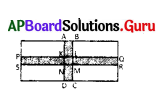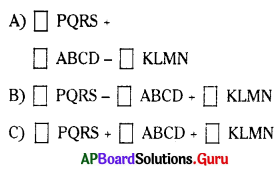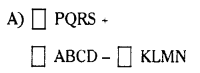Practice the AP 7th Class Maths Bits with Answers Chapter 13 Area and Perimeter on a regular basis so that you can attempt exams with utmost confidence.

## AP State Syllabus 7th Class Maths Bits 13th Lesson Area and Perimeter with Answers

Question 1.
The side of a square is 18cm. Then its area is ……….. cm2.
A) 36
B) 384
C) 324
D) 428
C) 324

Question 2.
The length and breadth of a rectangle are 25cm and 20cm then its perimeter is ……………. cm
A) 500
B) 90
C) 45
D) 100
B) 90Question 3.
The base of a parallelogram and its corresponding heights are 8cm and 3cm, its
area is …………….. cm2.
A) 12
B) 11
C) 48
D) 24
D) 24

Question 4.
Area of the given figure ……………. sq. units.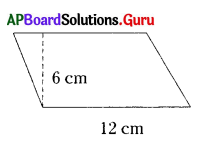A) 72
B) 36
C) 18
D) 48
A) 72

Question 5.
The base and height of a triangle are 10cm and 5cm, its area is
A) 50cm2
B) 25cm2
C) 30 cm2
D) 100cm2
B) 25cm2

Question 6.
In the figure BE = ………………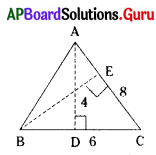A) 4
B) 5
C) 6
D) 3
D) 3

Question 7.
Area of the given figure is …………………. cm2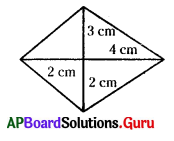A) 15
B) 30
C) 18
D) 24
A) 15

Question 8.
The diametre of a wheel is 35cm then its circumference is
A) 110cm
B) 220cm
C) 95cm
D) 100cm
A) 110cm

Question 9.
The radius of a circle is 7cm then its circumference is
A) 44cm
B) 36cm
C) 22 cm
D) 66cm
A) 44cmQuestion 10.
If the circumference is 22cm, then radius of the circle is
A) 1cm
B) 0.5cm
C) 3.5 cm
D) 14cm
C) 3.5 cm

Question 11.
The side of a square is 27 mts, then its area is ………….. sq. mts.
A) 729
B) 629
C) 829
D) 719
A) 729

Question 12.
If the side of a square is 7 cms, then its perimeter is ……………… cms.
A) 21
B) 14
C) 28
D) 35
C) 28

Question 13.
If the perimeter of a square is 36 cms, then its area is ………….. sq. cms.
A) 64
B) 81
C) 49
D) 18
B) 81

Question 14.
If l = 15 cm, b = 12 cm, then the perimeter of a rectangle is ……………… cms.
A) 180
B) 35
C) 27
D) 54
D) 54

Question 15.
From the above sum the area of a rectangle is ………………. sq. cm.
A) 150
B) 180
C) 160
D) 170
B) 180

Question 16.
If the area of a rectangle is 180 sq.cm its length is 20 cm, then its breadth is ……………. cm.
A) 9
B) 8
C) 7
D) 10
A) 9Question 17.
From the given figure, area of a parallelogram is …………….. sq. cm.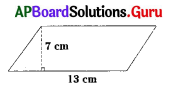A) 40.5
B) 81
C) 40
D) 91
D) 91

Question 18.
If the area of a parallelogram is 36 sq.cm, its base is 9 cm, then its height is ……….. cms.
A) 5
B) 6
C) 4
D) 3
C) 4

Question 19.
If the base of a parallelogram is twice of its height and area is 98 sq. cms, then its
base is ………… cms.
A) 16
B) 21
C) 7
D) 14
D) 14

Question 20.
From the given figure the area of a triangle is …………. sq. cms.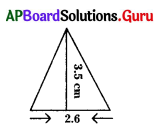A) 45.5
B) 4.55
C) 0.455
D) 5.55
B) 4.55

Question 21.
If the height of a triangle is 5 cm, and its area is 40 sq. cms, then its base is ………….. cms.
A) 16
B) 18
C) 20
D) 14
A) 16

Question 22.
From the given figure, $$\overline{\mathbf{B E}}$$ = ……………… cm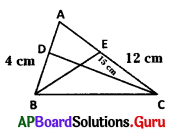A) 1
B) 5
C) 2
D) 4
B) 5

Question 23.
The base of a triangle is 40 cm and its area is 220 cm2, then its height is ……………….. cms.
A) 12
B) 11
C) 22
D) 14
B) 11Question 24.
The diagonals of a rhombus are (a + b), (a – b), then its area is …………….. sq. units
A) $$\frac {1}{2}$$ (2a)
B) $$\frac {1}{2}$$ (a2 + b2)
C) $$\frac {1}{2}$$ (a2 – b2)
D) $$\frac {1}{2}$$ (a + b) (2a)
C) $$\frac {1}{2}$$ (a2 – b2)

Question 25.
Find the area of a rhombus whose diagonals are 3 cm, 7 cm is …………….. sq. cms.
A) 10.5
B) 21
C) 10
D) 14
A) 10.5

Question 26.
The area of a rhombus is 96 cm2, its one of its diagonal length is 12 cm, then the length of second diagonal is ……………….. cm.
A) 24
B) 18
C) 16
D) 8
C) 16

Question 27.
The circumference of a circle whose radius is 3.5 cm is ……………….. cms.
A) 2.2
B) 44
C) 11
D) 22
D) 22

Question 28.
If the circumference of a circle is 55 cm, then its diameter is ………………. cm.
A) 18
B) 17.5
C) 17
D) 16.5
B) 17.5

Question 29.
If the circumference of a circle is 44 cm, then its diameter is …………… cms.
A) 16
B) 21
C) 7
D) 14
D) 14

Question 30.
The ratio of circumference of a circle to its diameter is
A) 7
B) 22
C) π
D) 7/22
C) πQuestion 31.
How many times will a wheel of radius 7 mts be rotated to travel 880 mts?
A) 30
B) 20
C) 25
D) 24
B) 20

Question 32.
How far does the tip of the minute hand move in 1 hour if its length is 14m is ………….. cms?
A) 88 cm
B) 80 cm
C) 98 cm
D) 78 cm
A) 88 cm

Question 33.
The area of a circle whose radius is 7 cm ……………..sq. cms.
A) 140
B) 176
C) 154
D) 166
C) 154

Question 34.
Which of the following is not true?
A) All parallelograms with equal base and equal heights are same area
B) Area of a parallelogram is related to area of a rectangle
C) A rectangle is a parallelogram
D) A parallelogram is a rectangle
D) A parallelogram is a rectangle

Question 35.
A square and a parallelogram have the same area. If a side of the square is 8 cm and the height of a parallelogram is 4 cm, then its base is ……………… cm.
A) 18
B) 16
C) 8
D) 32
B) 16

Question 36.
What is the area of a shaded region?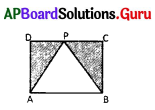A) Area of square ABCD – area of ΔAPB
B) Area of ΔABC – area of square ABCD
C) Area of square ABCD – area of [ΔAPD + ΔBPC]
D) None
A) Area of square ABCD – area of ΔAPB

Question 37.
Formula for area of a parallelogram is ……………… sq. units.
A) $$\frac {1}{2}$$ d (h1 + h2)
B) $$\frac {1}{2}$$ d1 d2
C) $$\frac {1}{2}$$ bh
D) bh
D) bh

Question 38.
Formula for area of a triangle is ………………. sq. units.
A) $$\frac {1}{2}$$ d (h1 + h2)
B) $$\frac {1}{2}$$ d1 d2
C) $$\frac {1}{2}$$ bh
D) bh
C) $$\frac {1}{2}$$ bhQuestion 39.
Area of a rhombus whose diagonals are d1, d2 is …………….
A) $$\frac {1}{2}$$ d (h1 + h2)
B) $$\frac {1}{2}$$ d1 d2
C) $$\frac {1}{2}$$ bh
D) bh
B) $$\frac {1}{2}$$ d1 d2

Question 40.
The area of the shaded path is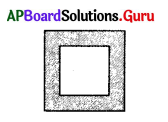A) Area of outer square – Area of inner square
B) Area of inner square – Area Of outer square
C) Area of outer square + Area of inner square
D) None
A) Area of outer square – Area of inner square

Question 41.
The circumference of a circle whose diameter is d units is given ………………
A) $$\frac{\pi \mathrm{d}}{2}$$
B) 2 π r
C) π d
D) $$\frac{\pi \mathrm{d}^{2}}{4}$$